Time: 3 Hours                                                                                                     Max. Marks: 100

NOTE: There are 9 Questions in all.

·      Question 1 is compulsory and carries 20 marks. Answer to Q. 1. must be written in the space provided for it in the answer book supplied and nowhere else.

·      Out of the remaining EIGHT Questions answer any FIVE Questions. Each question carries 16 marks.

·      Any required data not explicitly given, may be suitably assumed and stated.

Q.1       Choose the correct or best alternative in the following:                                         (2x10)

a.       A linear second order system with two complex poles in the left half of the s-plane is

(A)  over damped.                               (B)  critically damped.

(C)    under damped.                            (D)  undamped.

b.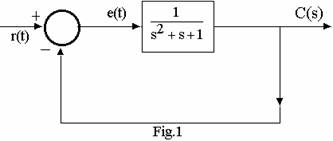In the system shown in Fig.1, for r(t) = a unit step function, the steady-state value of e(t) is

(A)    zero.

(B)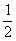.

(C)    1.

(D)    2.

c.   In the signal flow graph shown in Fig.2,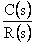is equal to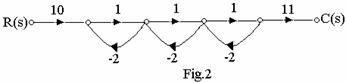(A)    10.

(B)     8.

(C)    6.

(D)    4.

d.   The open-loop transfer function of a feedback control system is given by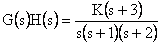, where K is a scalar gain parameter.  For large values of K, the root loci of the system are asymptotic to straight lines which intersect at a point whose coordinates in the s-plane are

(A)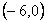(B)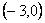(C)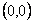(D)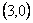e.   The response of an initially relaxed linear system for an input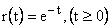is given by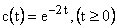.  The transfer function of the system is

(A)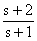(B)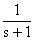(C)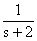(D)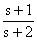f.    For studying the stability of a feedback control system that incorporates a dead-time (transportation lag) element, the most convenient method is

(A)     Routh-Hurwitz criterion                (B)  Nyquist criterion

(C)  Root locus                                    (D)  Bode plot

g.   An under damped linear second-order system is subjected to a step input signal.  The peak percentage overshoot in the response will depend upon

(A)     the natural frequency only.

(B)     the damping factor only.

(C)     both the natural frequency and the damping factor.

(D)    neither the natural frequency nor the damping factor.

h.   An ideal integrator with zero initial condition has a unit step input at time t=0.  The response of the integrator for t > 0 will be

(A)    1.                                                 (B) t.

(C)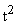.                                                (D)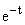.

i.    A second-order system has the transfer function G(s) given by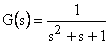.  The natural frequency and the damping factor of the system are respectively.

(A)   1 and 0.5.                                     (B) 1 and 2.

(C) 1 and 1.                                        (D) 2 and 1.

j.    The transfer function of a system is given by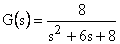then the impulse response of the system is given by

(A)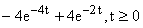.              (B)  constant,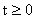.

(C)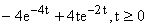.              (D)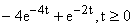.

Answer any FIVE Questions out of EIGHT Questions.

Each question carries 16 marks.

Q.2     a.   Consider the multiple-loop system shown in Fig.3.  Obtain the closed-loop transfer function between C(s) and N(s).                                             (10)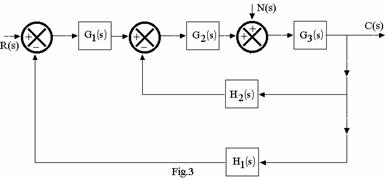b.   State and explain Mason’s Gain formula that gives the relationship between the input and output in a control system.                                                (6)

Q.3                                                                      a. Explain the working of a two-phase servomotor and give its torque-speed

characteristics.                                                    (8)

b.   Derive the transfer function of the electrical system shown in Fig.4.  Draw an schematic diagram of an equivalent mechanical system.              (8)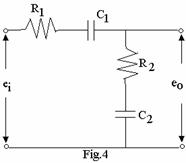Q.4     a.   Obtain the unit-step response of the system shown in Fig.5.                              (10)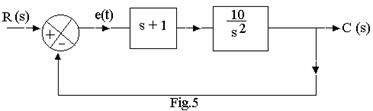b.   Explain the term, steady-state error?  Find the value of steady-state error in the system shown in Fig.5 for a unit step input.                                     (6)

Q.5     a.   Consider the closed-loop system given by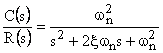Determine the values of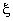and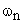so that the system responds to a step- input with approximately 5% over shoot and with settling time of                2 seconds. (Use the 2% criterion)                          (12)

b.   Explain the terms:

(i)  settling time.                                   (ii)  rise time.                                           (4)

Q.6           For the system shown in Fig.6, sketch the root loci for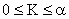.  Locate the closed-loop poles when the gain K is set equal to 2.                        (16)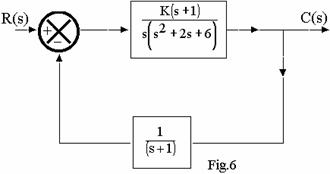Q.7     a.   A unity feedback control system is shown in Fig.7.  Determine the gain K such that the phase margin is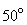.  What is the gain-margin in this case?     (10)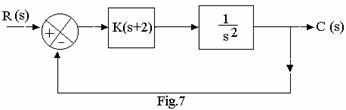b.   Examine the stability of the system shown in Fig.7, for K=1.                                (6)

Q.8           Write short notes on any TWO of the following:

(i)                  Phase-lag compensator.

(ii)                Synchro transmitter.

(iii)               LVDT.                                                                                    (16)

Q.9     a.   The characteristic equation of a feedback control system is given as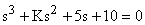Determine the value of K so that the system is marginally stable and the frequency of sustained oscillation, if applicable.                                           (10)

b.   Discuss the effects and limitations of phase-lead compensation.                           (6)